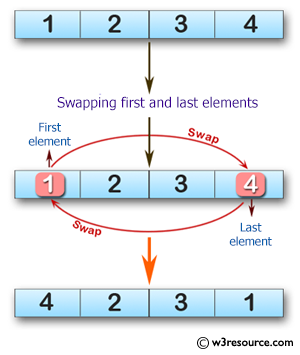﻿ Swift Array Programming Exercise: Swap the first and last elements of a given array of integers - w3resource# Swift Array Programming Exercises: Swap the first and last elements of a given array of integers

## Swift Array Programming: Exercise-19 with Solution

Write a Swift program to swap the first and last elements of a given array of integers. Return the modified array (length will be at least 1).

Pictorial Presentation:Sample Solution:

Swift Code:

`````` func swap_elements(_ a: [Int]) -> [Int] {
var new_array = a
let first_element = new_array.removeFirst()
let last_element = new_array.removeLast()
new_array.insert(last_element, at: new_array.startIndex)
new_array.append(first_element)

return new_array
}

print(swap_elements([1, 2, 3, 4]))
print(swap_elements([1, 2, 3]))
print(swap_elements([11, 12, 13, 14, 15]))
```
```

Sample Output:

```[4, 2, 3, 1]
[3, 2, 1]
[15, 12, 13, 14, 11]
```

Swift Programming Code Editor:

Improve this sample solution and post your code through Disqus

What is the difficulty level of this exercise?

﻿